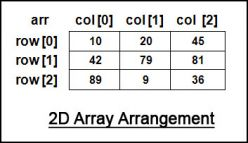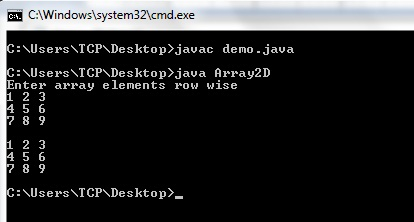# 2D Array in Java

In this tutorial you will learn about 2D array in Java. If
you don’t know about what is array then I would recommend you to read the
previous tutorial: Array in Java (1D)

## 2D Array in Java

Array is a collection of similar type of elements. Array can
be of any dimension but generally we don’t go beyond 3 dimensions. 2D array in
java is an array with two dimensions, rows and columns. 2D array is also known
as matrix.Image Source

### Declare 2D Array in Java

A 2 dimensional array can be declared in Java in following
two ways.
Syntax

Example

In above example I have declared reference variable that
will contain the reference of a 2D array object.

### Create 2D Array in Java

In Java, 2D array is created using new keyword in following
way.
Syntax

Example

In above example I have created a 2D array with 3 rows and 3
columns. This array can store 9 (3×3) elements.

### Declare, Create and Initialize 2D Array in Java

2D array can be declared, created and initialized simultaneously.
In this case we don’t need to write the new keyword. Take below example.

This will create a 2D array and initialize it. The above
array has 3 rows and 2 columns. To access the elements of 2D array we have to
use two indices, one for rows and another for columns.

### Program Example for 2D Array in Java

Lets make a simple program to create a 2D array, stores some
values in it and then print the values.

In this program I have used two for loops, the outer loop is
for rows and inner loop is for columns. I have initialized the array while
creating it. We can take the values from user also. Let’s make another program
that will read the values in array from user and then print it.In above program I have used Scanner class to read the values
from keyboard. nextInt() method is used to read integer values.

If you have any doubts related to above tutorial then feel
free to ask by commenting below.
Category: Java Array